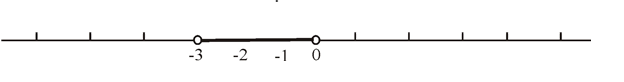# To express: the given interval in terms of an inequality and then graph the interval.### Precalculus: Mathematics for Calcu...

6th Edition
Stewart + 5 others
Publisher: Cengage Learning
ISBN: 9780840068071### Precalculus: Mathematics for Calcu...

6th Edition
Stewart + 5 others
Publisher: Cengage Learning
ISBN: 9780840068071

#### Solutions

Chapter 1.1, Problem 45E
To determine

## To express: the given interval in terms of an inequality and then graph the interval.

Expert Solution

In terms of inequality the interval can be expressed as 3<x<0 .

### Explanation of Solution

Given information:

An interval is given as

(3,0)

Concept used:

An interval (a,b) in terms of an inequality can be expressed as a<x<b .

An interval [a,b] in terms of an inequality can be expressed as axb .

An interval [a,b) in terms of an inequality can be expressed as ax<b .

An interval (a,b] in terms of an inequality can be expressed as a<xb .

Calculation:

Consider the given interval.

(3,0)

Let us consider a variable x o express the inequality.

So, the interval can be expressed as an inequality as 3<x<0 .

Graph:

Now, graph the interval on number line as shown:Here, 3and0 both the points are excluded from the interval so, open balls are placed for 3and0 on the number line.

### Have a homework question?

Subscribe to bartleby learn! Ask subject matter experts 30 homework questions each month. Plus, you’ll have access to millions of step-by-step textbook answers!